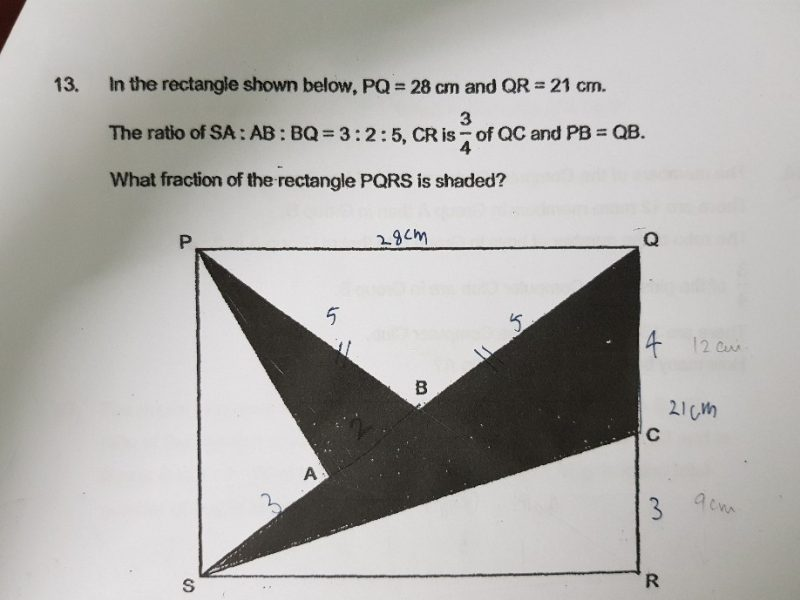# QuestionHi Kaasu Parents,

Thank you,

DNLNMUM

you have labelled the ratio, so

Area of ΔQSC: Area of ΔSCR = 4:3 ( if you can get this part, you almost get the answer)

so Area of ΔQSC = 4/7 of ΔQSR = 2/7 of rectangle PQRS

second, Area of ΔPAB = 2/10 of  ΔPSQ = 1/10 of rectangle PQRS

so the total shaded area is 2/7+ 1/10 = 27/70 of rectangle PQRS